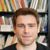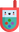## Julia Community 🟣

Vinod V

Posted on • Updated on • Originally published at pythonjulia.blogspot.com

# Idempotent matrices in Julia

Let $A$ be a square matrix of order $n$ . Then $A$ is called an idempotent matrix if $AA = A$ .

If a matrix $A$ is idempotent, it follows that $A^n = A, \forall n \in \mathbb{N}$ . All Idempotent matrices except identity matrices are singular matrices.

One way to make idempotent matrices is $A = I - u u^T$ , where $u$ is a vector satisfying $u^T u = 1$ . In this case $A$ is symmetric too.

#Idempotent matrices in Julia
using LinearAlgebra
u = rand(5)
u = u/norm(u) #Force u^T * u = 1
A = I - u*u'
isapprox(A^100,A) # true
isequal(A,A') # true (test for symmetricity)Logan Kilpatrick

Hey! This is cool to see, it would be nice to also add some context for folks about why you would need this, etc etc. You can also set the canonical URL if you edit this post to point to the original blog post above.Comment deletedComment deletedComment deleted